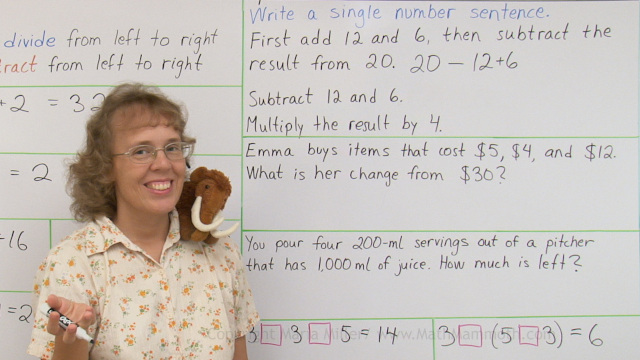^

Find here free online math videos topics related to addition and subtraction, meant for 1st, 2nd, 3rd, and 4th grades.

These videos match the topics in Math Mammoth Addition 1 (Blue Series).

Learn the symbols + and =

Addition facts within 10 — six videos matching the lessons titled "Sums with 6", "Sums with 7", "Sums with 8", "Sums with 9", and "Sums with 10".

Comparisons – compare numbers and sums

## Subtraction within 0-10 and related concepts

These videos match the topics in Math Mammoth Subtraction 1 worktext (Blue Series).

Subtraction and Addition in the Same Picture – the connection between subtraction and addition

Fact families

The concept of "how many more"

"How many more" problems and differences

Subtract many numbers

## Addition & subtraction facts within 0-10

These videos match the topics in Math Mammoth Subtraction 1 worktext (Blue Series).

Addition and subtraction facts with 6 (fact families)

Addition and subtraction facts with 7 (fact families)

Addition and subtraction facts with 8 (fact families)

Addition and subtraction facts with 9 (fact families)

## Addition and Subtraction Facts Within 0-18

These videos match the topics in Math Mammoth Add & Subtract 2-A worktext (Blue Series).

A mental math trick with nine and eight

Difference, subtraction, and "How many more" additions

Number rainbow & fact families with 11

Number rainbow & fact families with 12

Number rainbow & fact families with 13

Number rainbow & fact families with 14

## Mental addition and subtraction of two-digit numbers

These videos match the topics in Math Mammoth Add & Subtract 2-B worktext (Blue Series).

Adding within the same ten – add a 2-digit number and a single-digit number mentally - no regrouping

Add and subtract two-digit numbers – without regrouping

Even & odd numbers

Doubling

One-half of a number

Pictographs

Add and subtract whole tens (to/from 2-digit numbers)

Going over ten — "make ten" (and go over ten) strategy for addition

## Regrouping in addition and subtraction (2-digit numbers)

These videos match the topics in Math Mammoth Add & Subtract 2-B worktext (Blue Series).

Add a 2-digit number and a single-digit number mentally – no regrouping

Regrouping with tens

Add three or four single-digit numbers mentally

Regrouping in subtraction, parts 1 and 2 (with 2-digit numbers)

Regrouping in subtraction, part 3 – includes word problems with Mathy and Giganto (mammoth mascots)

Mental subtraction, parts 1 and 2 – strategies for mental subtraction

## Regrouping in Addition and Subtraction with 3-digit numbers

These videos match the topics in Math Mammoth Add & Subtract 3 worktext (Blue Series).

Regrouping 10 tens as one hundred (3-digit addition)

Regrouping one hundred as 10 tens in subtraction

Regrouping one hundred as 10 tens in subtraction: word problems and puzzles

Regrouping twice in subtraction

The connection with addition and subtraction

Mileage chart

The order of operations — parenthesis, addition & subtraction only

These videos match the topics in Math Mammoth Add & Subtract 4 worktext (Blue Series).

Bar models in addition and subtraction problemsThe order of operations and writing simple expressions

Making a bar graph plus word problems

Rounding 4-digit numbers

Estimating the final result

Calculate and estimate money amounts

Calculate and estimate money amounts: word problems

WAIT!

Receive my monthly collection of math tips & resources directly in your inbox — and get a FREE Math Mammoth book!You can unsubscribe at any time.

### Math Mammoth Tour

Confused about the different options? Take a virtual email tour around Math Mammoth! You'll receive:

An initial email to download your GIFT of over 400 free worksheets and sample pages from my books. Six other "TOURSTOP" emails that explain the important things and commonly asked questions concerning Math Mammoth curriculum. (Find out the differences between all these different-colored series!)

This way, you'll have time to digest the information over one or two weeks, plus an opportunity to ask me personally about the curriculum.
A monthly collection of math teaching tips & Math Mammoth updates (unsubscribe any time)### "Mini" Math Teaching Course

This is a little "virtual" 2-week course, where you will receive emails on important topics on teaching math, including:

- How to help a student who is behind
- Troubles with word problems
- Teaching multiplication tables
- Why fractions are so difficult
- The value of mistakes
- Should you use timed tests
- And more!

A GIFT of over 400 free worksheets and sample pages from my books right in the very beginning.A monthly collection of math teaching tips & Math Mammoth updates (unsubscribe any time)
Enter your email to receive math teaching tips, resources, Math Mammoth news & sales, humor, and more! I tend to send out these tips about once monthly, near the beginning of the month, but occasionally you may hear from me twice per month (and sometimes less often).• A GIFT of over 400 free worksheets and sample pages from my books.